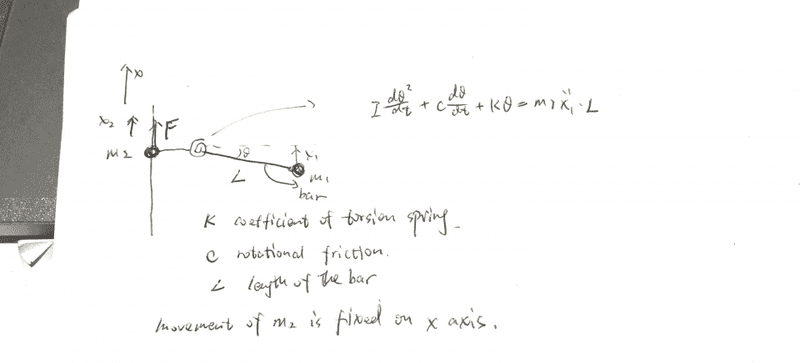Here I want to analyse the force imposed on m1 and m2 while using the second Newton law.
The top view of the system shows like below, thus gravity force is not considered, m2 can only move along the vertical line, and one torsion spring link m1 and m2. The length between m1 and spring is L, length between m2 and spring is negligible. The I have written the equation related to spring. where x1 and x2 is the position of m1 and m2 respectively. F is the force on m2.

My question is how should I write a equation related to x2 and force F. And equation about the whole system. Thank you.haruspex
Homework Helper
Gold Member
2020 Award
Two questions...
Why the dθ/dt factor in the frictional term? Isn't torsional friction just a constant, so long as the motion is in one direction?
If m2 is accelerating, shouldn't that contribute to m1's acceleration? I.e., shouldn't the right hand side be ##m_1(\ddot x_1-\ddot x_2)L##?

Thank you for your reply. The m2 can move upwards and downwards, in two direction. The second question is what I concerned. I don't know how to write the equation to represent this.

haruspex